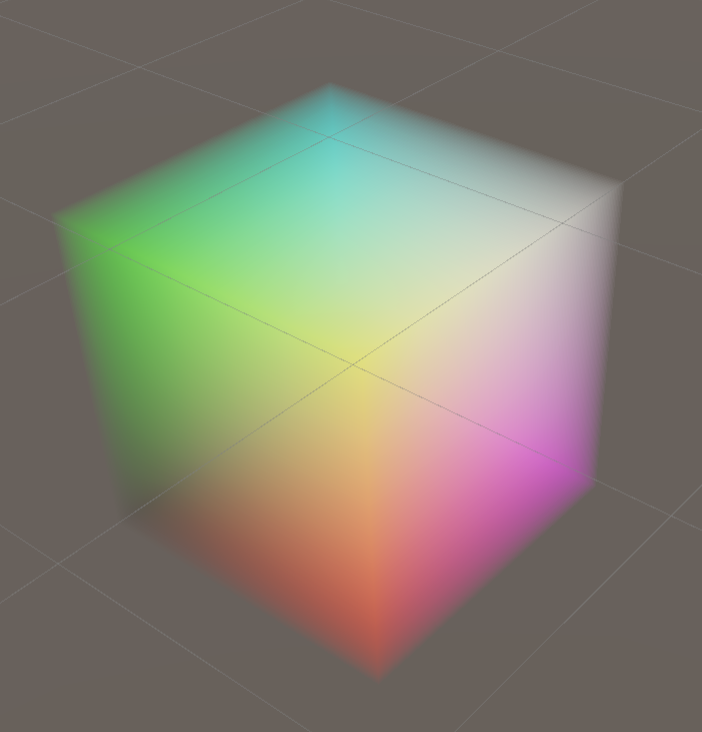Version: 2019.3
Language : English
Movie Textures
Texture arrays

# 3D textures

Switch to Scripting

A 3D texture is a bitmap image that contains information in three dimensions rather than the standard two. 3D textures are commonly used to simulate volumetric effects such as fog or smoke, to approximate a volumetric 3D , or to store animated textures and blend between them smoothly.

In your Unity Project, the Unity Editor represents 3D textures as Texture Assets. To configure a Texture Asset’s import settings you can select the Texture Asset and use the , or write a script that uses the TextureImporter API.

In the Unity engine, Unity uses the Texture3D class to represent 3D textures. Use this class to interact with 3D textures in C# .

## 3D texture size

The maximum resolution of a 3D texture is 2048 x 2048 x 2048.

Be aware that the size of a 3D texture in memory and on disk increases quickly as its resolution increases. An RGBA32 3D texture with no mip maps and a resolution of 16 x 16 x 16 has a size of 128KB, but with a resolution of 256 x 256 x 256 it has a size of 512MB.

## Creating a 3D texture

To create a 3D texture in your Project, you must use a script.

The following example is an Editor script that creates an instance of the Texture3D class, populates it with color data, and then saves it to your Project as a Texture Asset.

``````using UnityEditor;
using UnityEngine;

public class ExampleEditorScript : MonoBehaviour
{
[MenuItem("CreateExamples/3DTexture")]
static void CreateTexture3D()
{
// Configure the texture
int size = 32;
TextureFormat format = TextureFormat.RGBA32;
TextureWrapMode wrapMode =  TextureWrapMode.Clamp;

// Create the texture and apply the configuration
Texture3D texture = new Texture3D(size, size, size, format, false);
texture.wrapMode = wrapMode;

// Create a 3-dimensional array to store color data
Color[] colors = new Color[size * size * size];

// Populate the array so that the x, y, and z values of the texture will map to red, blue, and green colors
float inverseResolution = 1.0f / (size - 1.0f);
for (int z = 0; z < size; z++)
{
int zOffset = z * size * size;
for (int y = 0; y < size; y++)
{
int yOffset = y * size;
for (int x = 0; x < size; x++)
{
colors[x + yOffset + zOffset] = new Color(x * inverseResolution,
y * inverseResolution, z * inverseResolution, 1.0f);
}
}
}

// Copy the color values to the texture
texture.SetPixels(colors);

// Apply the changes to the texture and upload the updated texture to the GPU
texture.Apply();

// Save the texture to your Unity Project
AssetDatabase.CreateAsset(texture, "Assets/Example3DTexture.asset");
}
}
``````

## Using a 3D texture in a shader

Here is an example of a simple raymarching that uses a 3D texture to visualize a volume.

``````Shader "Unlit/VolumeShader"
{
Properties
{
_MainTex ("Texture", 3D) = "white" {}
_Alpha ("Alpha", float) = 0.02
_StepSize ("Step Size", float) = 0.01
}
SubShader
{
Tags { "Queue" = "Transparent" "RenderType" = "Transparent" }
Blend One OneMinusSrcAlpha
LOD 100

Pass
{
CGPROGRAM
#pragma vertex vert
#pragma fragment frag

#include "UnityCG.cginc"

// Maximum amount of raymarching samples
#define MAX_STEP_COUNT 128

// Allowed floating point inaccuracy
#define EPSILON 0.00001f

struct appdata
{
float4 vertex : POSITION;
};

struct v2f
{
float4 vertex : SV_POSITION;
float3 objectVertex : TEXCOORD0;
float3 vectorToSurface : TEXCOORD1;
};

sampler3D _MainTex;
float4 _MainTex_ST;
float _Alpha;
float _StepSize;

v2f vert (appdata v)
{
v2f o;

// Vertex in object space this will be the starting point of raymarching
o.objectVertex = v.vertex;

// Calculate vector from camera to vertex in world space
float3 worldVertex = mul(unity_ObjectToWorld, v.vertex).xyz;
o.vectorToSurface = worldVertex - _WorldSpaceCameraPos;

o.vertex = UnityObjectToClipPos(v.vertex);
return o;
}

float4 BlendUnder(float4 color, float4 newColor)
{
color.rgb += (1.0 - color.a) * newColor.a * newColor.rgb;
color.a += (1.0 - color.a) * newColor.a;
return color;
}

fixed4 frag(v2f i) : SV_Target
{
// Start raymarching at the front surface of the object
float3 rayOrigin = i.objectVertex;

// Use vector from camera to object surface to get ray direction
float3 rayDirection = mul(unity_WorldToObject, float4(normalize(i.vectorToSurface), 1));

float4 color = float4(0, 0, 0, 0);
float3 samplePosition = rayOrigin;

// Raymarch through object space
for (int i = 0; i < MAX_STEP_COUNT; i++)
{
// Accumulate color only within unit cube bounds
if(max(abs(samplePosition.x), max(abs(samplePosition.y), abs(samplePosition.z))) < 0.5f + EPSILON)
{
float4 sampledColor = tex3D(_MainTex, samplePosition + float3(0.5f, 0.5f, 0.5f));
sampledColor.a *= _Alpha;
color = BlendUnder(color, sampledColor);
samplePosition += rayDirection * _StepSize;
}
}

return color;
}
ENDCG
}
}
}
``````

If you use this shader with the 3D texture created in the example at the top of the page, the result looks like this:Movie Textures
Texture arrays